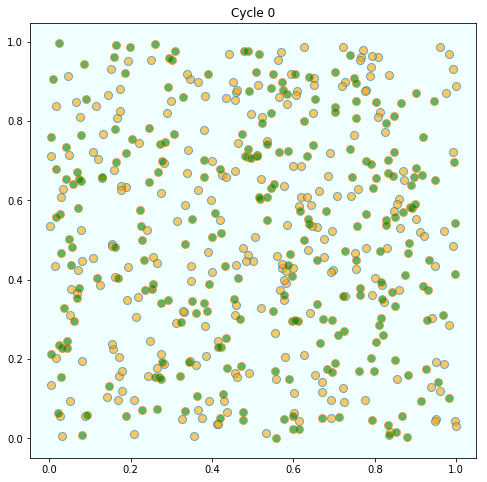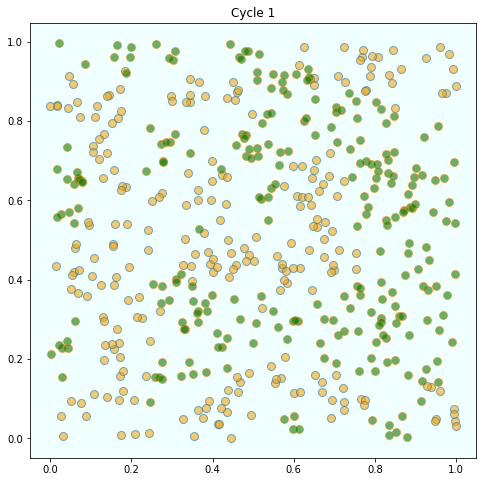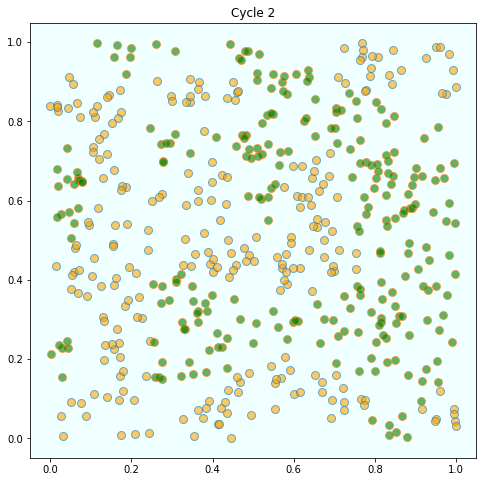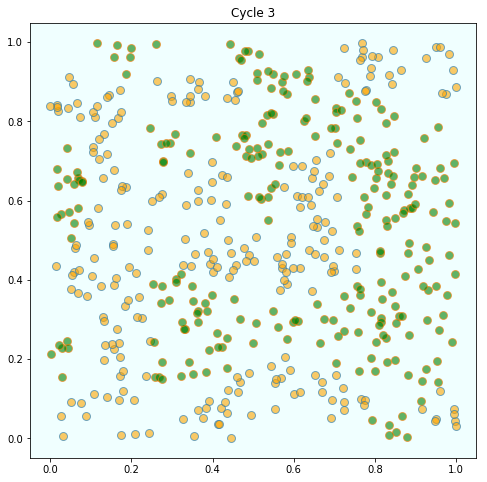We are working to support a site-wide PDF but it is not yet available. You can download PDFs for individual lectures through the download badge on each lecture page.

Code should execute sequentially if run in a Jupyter notebook

Schelling’s Segregation Model¶

Outline¶

In 1969, Thomas C. Schelling developed a simple but striking model of racial segregation [Sch69]

His model studies the dynamics of racially mixed neighborhoods

Like much of Schelling’s work, the model shows how local interactions can lead to surprising aggregate structure

In particular, it shows that relatively mild preference for neighbors of similar race can lead in aggregate to the collapse of mixed neighborhoods, and high levels of segregation

In recognition of this and other research, Schelling was awarded the 2005 Nobel Prize in Economic Sciences (joint with Robert Aumann)

In this lecture we (in fact you) will build and run a version of Schelling’s model

The Model¶

We will cover a variation of Schelling’s model that is easy to program and captures the main idea

Set Up¶

Suppose we have two types of people: orange people and green people

For the purpose of this lecture, we will assume there are 250 of each type

These agents all live on a single unit square

The location of an agent is just a point $(x, y)$, where $0 < x, y < 1$

Preferences¶

We will say that an agent is happy if half or more of her 10 nearest neighbors are of the same type

Here ‘nearest’ is in terms of Euclidean distance

An agent who is not happy is called unhappy

An important point here is that agents are not averse to living in mixed areas

They are perfectly happy if half their neighbors are of the other color

Behavior¶

Initially, agents are mixed together (integrated)

In particular, the initial location of each agent is an independent draw from a bivariate uniform distribution on $S = (0, 1)^2$

Now, cycling through the set of all agents, each agent is now given the chance to stay or move

We assume that each agent will stay put if they are happy and move if unhappy

The algorithm for moving is as follows

1. Draw a random location in $S$
2. If happy at new location, move there
3. Else, go to step 1

In this way, we cycle continuously through the agents, moving as required

We continue to cycle until no one wishes to move

Results¶

Let’s have a look at the results we got when we coded and ran this model

As discussed above, agents are initially mixed randomly togetherBut after several cycles they become segregated into distinct regionsIn this instance, the program terminated after 4 cycles through the set of agents, indicating that all agents had reached a state of happiness

What is striking about the pictures is how rapidly racial integration breaks down

This is despite the fact that people in the model don’t actually mind living mixed with the other type

Even with these preferences, the outcome is a high degree of segregation

Exercises¶

Exercise 1¶

Implement and run this simulation for yourself

Consider the following structure for your program

Agents can be modeled as objects

Here’s an indication of how they might look

* Data:

* type (green or orange)
* location

* Methods:

* Determine whether happy or not given locations of other agents

* If not happy, move

* find a new location where happy


And here’s some pseudocode for the main loop

while agents are still moving
for agent in agents
give agent the opportunity to move


Use 250 agents of each type

Solutions¶

Exercise 1¶

Here’s one solution that does the job we want

If you feel like a further exercise you can probably speed up some of the computations and then increase the number of agents

In :
from random import uniform, seed
from math import sqrt
import matplotlib.pyplot as plt
%matplotlib inline

seed(10)  # for reproducible random numbers

class Agent:

def __init__(self, type):
self.type = type
self.draw_location()

def draw_location(self):
self.location = uniform(0, 1), uniform(0, 1)

def get_distance(self, other):
"Computes euclidean distance between self and other agent."
a = (self.location - other.location)**2
b = (self.location - other.location)**2
return sqrt(a + b)

def happy(self, agents):
"True if sufficient number of nearest neighbors are of the same type."
distances = []
# distances is a list of pairs (d, agent), where d is distance from
# agent to self
for agent in agents:
if self != agent:
distance = self.get_distance(agent)
distances.append((distance, agent))
# == Sort from smallest to largest, according to distance == #
distances.sort()
# == Extract the neighboring agents == #
neighbors = [agent for d, agent in distances[:num_neighbors]]
# == Count how many neighbors have the same type as self == #
num_same_type = sum(self.type == agent.type for agent in neighbors)
return num_same_type >= require_same_type

def update(self, agents):
"If not happy, then randomly choose new locations until happy."
while not self.happy(agents):
self.draw_location()

def plot_distribution(agents, cycle_num):
"Plot the distribution of agents after cycle_num rounds of the loop."
x_values_0, y_values_0 = [], []
x_values_1, y_values_1 = [], []
# == Obtain locations of each type == #
for agent in agents:
x, y = agent.location
if agent.type == 0:
x_values_0.append(x)
y_values_0.append(y)
else:
x_values_1.append(x)
y_values_1.append(y)
fig, ax = plt.subplots(figsize=(8, 8))
plot_args = {'markersize': 8, 'alpha': 0.6}
ax.set_facecolor('azure')
ax.plot(x_values_0, y_values_0, 'o', markerfacecolor='orange',  **plot_args)
ax.plot(x_values_1, y_values_1, 'o', markerfacecolor='green', **plot_args)
ax.set_title(f'Cycle {cycle_num-1}')
plt.show()

# == Main == #

num_of_type_0 = 250
num_of_type_1 = 250
num_neighbors = 10      # Number of agents regarded as neighbors
require_same_type = 5   # Want at least this many neighbors to be same type

# == Create a list of agents == #
agents = [Agent(0) for i in range(num_of_type_0)]
agents.extend(Agent(1) for i in range(num_of_type_1))

count = 1
# ==  Loop until none wishes to move == #
while True:
print('Entering loop ', count)
plot_distribution(agents, count)
count += 1
no_one_moved = True
for agent in agents:
old_location = agent.location
agent.update(agents)
if agent.location != old_location:
no_one_moved = False
if no_one_moved:
break

print('Converged, terminating.')

Entering loop  1Entering loop  2Entering loop  3Entering loop  4Converged, terminating.

• Share page